Importance: Medium ✭✭
 Author(s): Feige, Uriel
 Subject: Theoretical Computer Science
 Keywords: Inequality Probability Theory randomness in TCS
 Posted by: cwenner on: April 2nd, 2009
Conjecture   Ifare independent random variables with, then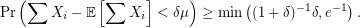In comparison to most probabilistic inequalities (like Hoeffding's), Feige's inequality does not deteriorate asgoes to infinity, something that is useful for computer scientists.

Let. Feige argued that to prove the conjecture, one only needs to prove it for the case when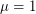and each variablehas the entire probability mass distributed on 0 and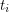for some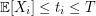. He proved that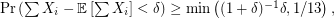and conjectured that the constant 1/13 may be replaced with. It was further conjectured that "the worst case" would be one of

\item one variable has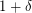as maximum value and the remaining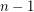random variables are always 1 (hence the probability that the sum is less thanis), \item each variable has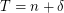as maximum (hence the probability that the sum is less thanis).

One way to initiate an attack on this problem is to assumeand argue that the case when each variable assumeswith probabilityand otherwise 0 is indeed the worst.

## Bibliography

*[F04] Uriel Feige: On sums of independent random variables with unbounded variance, and estimating the average degree in a graph, STOC '04: Proceedings of the thirty-sixth annual ACM symposium on Theory of computing (2004), pp. 594 - 603. ACM

*[F05] Uriel Feige: On sums of independent random variables with unbounded variance, and estimating the average degree in a graph, Manuscript, 2005, [pdf]

The problem was also referenced at population algorithms, the blog.

* indicates original appearance(s) of problem.